# Chern class

## Definition

The Chern class is a characteristic class (or collection of characteristic classes in different dimensions) for the topological group$GL(n,\mathbb{C})$ with coefficients in$\mathbb{Z}$.

Axiomatically, the Chern class can be defined as associating to every complex vector bundle$p:E \to B$ a class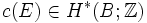$c(E) \in H^*(B;\mathbb{Z})$ which lives only in even degrees, such that if$c_i(E)$ denotes the component of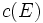$c(E)$ in the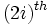$(2i)^{th}$ graded component, the following hold:

1.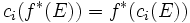$c_i(f^*(E)) = f^*(c_i(E))$ (this is the condition for being a natural transformation, part of the definition of characteristic class)
2.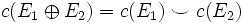$c(E_1 \oplus E_2) = c(E_1) \smile c(E_2)$ where$\smile$ denotes the cap product. This is a Whitney sum formula
3.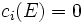$c_i(E) = 0$ if$i$ is greater than the dimension of$E$
4. For the canonical complex line bundle$E \to \mathbb{C}P^\infty$,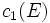$c_1(E)$ is a pre-specified generator of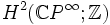$H^2(\mathbb{C}P^\infty; \mathbb{Z})$$c$ is termed the total Chern class and$c_i$ is termed the$i^{th}$ Chern class.